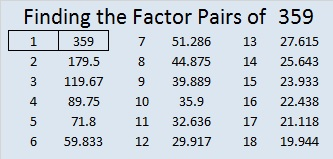# 359 and Level 2

When 2^359 is divided by 359, the remainder is 2, so 359 is VERY LIKELY a prime number. Scroll down past the puzzle to know for sure.Print the puzzles or type the factors on this excel file: 10 Factors 2015-01-19

• 359 is a prime number.
• Prime factorization: 359 is prime.
• The exponent of prime number 359 is 1. Adding 1 to that exponent we get (1 + 1) = 2. Therefore 359 has exactly 2 factors.
• Factors of 359: 1, 359
• Factor pairs: 359 = 1 x 359
• 359 has no square factors that allow its square root to be simplified. √359 ≈ 18.947How do we know that 359 is a prime number? If 359 were not a prime number, then it would be divisible by at least one prime number less than or equal to √359 ≈ 18.947. Since 359 cannot be divided evenly by 2, 3, 5, 7, 11, 13, or 17, we know that 359 is a prime number.This site uses Akismet to reduce spam. Learn how your comment data is processed.